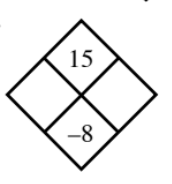### Home > MC1 > Chapter 5 > Lesson 5.1.2 > Problem5-21

5-21.

Copy and complete each of the Diamond Problems below. The pattern used in the Diamond Problems is shown at right.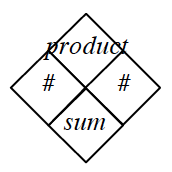1.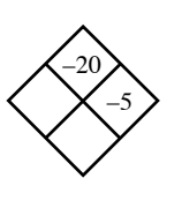What number multiplied by $−5$ is equal to $−20$? Add the number you just got to $−5$ in order to fill the sum box.

See the diamond below.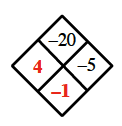1.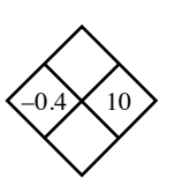Since you have both numbers, you just have to multiply them and add them to fill the other boxes.

1.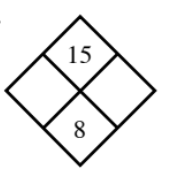For parts (c) and (d), think about what two numbers could multiply to the given product and add to the given sum.
These numbers will fill the empty boxes.

1.